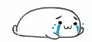# 关于OI中简单的常数优化

1.IO(istream/ostream) 输入输出优化

输入：

$1.cin$ 呵呵，不说什么了，慢的要死。大概$1e8$个数要读1分钟左右

$2.scanf, \_ \_ builtin \_ scanf()$ $scanf$ 其实还不算太快，但是$\_ \_ builtin \_$在$NOIp$赛场上会$CE$

$3.read()$ 美其名曰：读入优化(反正本宝宝不会)，在各大神犇的提交记录以及题解上随处可见，亲测确实比$scanf$要快许多

代码大概长这样: $by\ Young\ Neal$

int getint(){
int x=0,f=0;char ch=getchar();
while(!isdigit(ch)) f|=ch=='-',ch=getchar();
while(isdigit(ch)) x=(x<<1)+(x<<3)+(ch^48),ch=getchar();
return f?-x:x;
}

$4. fread$ 欢迎来自$AK$爷的飞速文件读入输出

struct file_io{
#define isdigit(ch) ((ch) >= '0' && (ch) <= '9')
char inbuf[1 << 25], *pin, outbuf[1 << 25], *pout;
int stk;

file_io(): pout(outbuf) {fread(pin = inbuf, 1, 1 << 25, stdin);}
~file_io() {fwrite(outbuf, 1, pout - outbuf, stdout);}

inline void getint(int &num){
bool neg = 0; num = 0;
while(!isdigit(*pin)) if(*pin++ == '-') neg = 1;
while(isdigit(*pin)) num = num * 10 + *pin++ - '0';
if(neg) num = -num;
}

inline void putint(int num){
static int *v = stk;
if(!num) *pout++ = '0';
else{
if(num < 0) *pout++ = '-', num = -num;
for(; num; num /= 10) *v++ = num % 10;
while(v != stk) *pout++ = *--v + '0';
}
}

inline void nextline() {*pout++ = '\n';}
} fio;
#define getint(num) fio.getint(num)
#define putint(num) fio.putint(num)
#define nextline() fio.nextline()

输出：

$1.cout$ 呵呵，和$cin$一个样

$2.printf , \_ \_ builtin \_ printf$ 已经比上面那个快多了，但是一样，$\_ \_ builtin \_ printf$会$CE$

$3.puts("")$ 对于已知字符串来说，能用 $puts()$不用$printf()$ 但是$puts("")$输出之后会换行

$4.fio$见上面 (STO GhostCai)2.内存优化

某$shadowice1984$大佬最擅长的东东，以下引用$shadowice1984$给本宝宝讲课时候的话：

“我们的$CPU$要对某一个数进行计算的时候，会先在一级缓存中找这个数的地址要是找到了，直接揪过来~~枪毙~~进行计算，速度很快的，

当本宝宝听完这段话之后，$1.$保证内存的连续访问

这就是为什么本宝宝邻接链表写的每次都比别人慢上差不多一倍$qwq$ ，因为现在只有本宝宝用结构体写了啊啊啊啊啊啊！

$2.$多个$for$循环可以适当调整顺序

最明显的就是矩阵乘法和$Folyd$算法了，对于$folyd$ $(eg. \ NOIp2016 \text{换教室})$

for(int i=1;i<=n;i++)
for(int j=1;j<i;j++)
for(int k=1;k<=n;k++)
d[i][j]= d[j][i]=min(d[i][j],d[i][k]+d[k][j]);

for(int k=1;k<=n;k++)
for(int i=1;i<=n;i++)
for(int j=1;j<i;j++)
d[i][j]=d[j][i]=min(d[i][j],d[i][k]+d[k][j]);

亲测第二种写法会比第一种写法快好多呢。

矩阵乘法同理，

for(int k=1;k<=n;k++)
for(int i=1;i<=n;i++)
for(int j=1;j<=n;j++)
c.num[i][j]+=a.num[i][k]*b.num[k][j];

把$k$这一维放到外面明显比放到里面要快

3.register 与 inline

$register$在$for$里面真的会快一点，用法就是$for(register int i=1;i<=n;i++)$

$inline$ 只能用在没有递归的函数里，其实手动$inline$是个很好的东西，但是不一定。

这两个有的时候会造成负优化，这就呵呵了。4.(仅限于$NOIp$等不开$O2$的赛事上) 手写$stl$

$stl(\ C++ \ Standard\ Template \ Library \ )$  确实特别好用，也是$C++$的精华所在，

可以为程序员们节省很大的时间，

但是，在不开$O2$的情况下，会因为种种原因慢的要死，

尽量背过一些简单的数据结构，能少用就少用。

5.玄学（信仰）优化：

(1). 打表优化

如果有的写的非正解，但是又想拿高分，全打表得话会超过代码长度限制，这是后可以部分打表，记得做过一道题

对于30%的数据，满足n<=500;

对于100%的数据，满足n<=1000;

然后我和$shadowice1984$都会一个$On^{3}$的方法，显然肯定过不了$1000$

之后，本宝宝放弃了，拿了$30$分去一边哭去了，

$shadowice1984$，$n^{3}$信仰过$500$，然后又信仰的打了一下$n=995$到$n=1000$的表

然后就$……$ 然后就 $A$ 了数据范围内，只打极限数据，放心，出题人一定会很毒瘤的

还有优化打表的方法：查分优化，二次查分优化，$26$进制压缩（$eg.$树的平均路长问题）

(2).女装优化(亲测有效)

女装可以大大的减少$bug$和常数，真的亲测有效，模拟赛的时候$t$的东西，**后再测就过了。。

(3).神犇优化(亲身经历)

有一次网上打$nowcoder$的比赛，然后和旁边的神犇代码比较一下只有变量名不同(才不是互相抄的呢)

然后$……$人家神犇就过了，本宝宝就$T$了，

平时做题的时候，本宝宝经常出现用时和神犇差距很大，

自己写线段树都已经不记录$l,r$了，还比那些开结构体记录$l,r$的神犇慢$……$

真的是人菜常数大，真的是$……$

~~所以想暴力碾标算的话，先要成为神犇~~(4).信仰优化

$srand(1926****)$ $srand(\text{cp或神犇生日})$

$eg.\ srand(20020902) \ \ srand(20020224)$

(左cp右神犇)

然后当你

printf("%s",rand()%2?"Yes":"No")posted @ 2018-08-02 11:32  米罗偕涯  阅读(2471)  评论(12编辑  收藏  举报